### The Lorentz transformation

#### Problem:

In the year 2310 construction of the large space colony "Heavy Metal" is finally completed.  The distance between the "East" and "West" edges of the colony is 400 km in the rest frame of the colony.  To celebrate the end of construction, two flares are lighted at midnight during a New Year's celebration at the "East" and "West" edges.  The light from the flares reaches an observer at the center of the colony at the same time.  A fast spacecraft flying with a constant speed of v = 0.95 c "East" to "West".  Let Event 1 be flare is lighted on "East" edge and Event 2 be flare is lighted on "West" edge.  In the reference frame of the spacecraft, does Event 1 occur before, after, or at the same time as Event 2?  Explain your reasoning!

Solution:

• Concepts:
The Lorentz transformation, the simultaneity problem
• Reasoning:
We are given the space-time coordinates of two events in one reference frame.  We are asked to transform to another reference frame.
• Details of the calculation:
Let the x axis point from "East" to "West".
Put the origins of the coordinate systems of the colony and the spacecraft at the "East" edge and synchronizes the clocks at t = 0 when the flare is lighted on "East" edge.  Event 1 has coordinates x = 0, t = 0 in both frames.  Event 2 has coordinates x = 400 km, t = 0 in the rest frame of the colony.
To find the time t' the flare is lighted on the "West" edge in the frame of the galaxy we use ct' = γct - γβx = - γβ(400 km).
t' is negative, Event 2 occurred before event 1 in the frame of the spaceship.

#### Problem:

A space traveler, moving with velocity vi with respect to Earth synchronizes his clock with a friend on Earth at t' = t = 0.  The earthman then observes both clocks and simultaneously reads the times t and t' (t directly and t' through a telescope).  What does t read when t' reads 1 hour?

Solution:

• Concepts:
Lorentz transformation
• Reasoning:
If in the spaceship the coordinates of an event are t' and x' = 0, then the coordinates of the same events on earth are t = γt', x = γvt'.
• Details of the calculation:
The light signal from this event reaches earth after a time interval ∆t = x/c = γβt'.
The earth clock will read t + ∆t = γ(1 + β)t' = [(c + v)/(c - v)]½*(1hour) when the earthman sees t' = 1 hour.

#### Problem:

For an observer on Earth, the star Sirius is 8.8 ly away.  Assume Sirius is stationary with respect to Earth.  At t = 0, a spaceship moving with velocity v = 0.95 c i towards Sirius passes Earth.  Earth and the ship synchronize clocks.
An observer on Earth tells the following story at a much later time:
At t = 0, just as the spaceship passed Earth, a flare erupted on Sirius.  The light from the flare reached Earth at t = 8.8 y.  At t = 0.9 years a comet hit Earth.  At t = 9.26 years the spaceship passed Sirius and sent us a light signal.  The signal reached us at 18.06 years.
How does an observer on the ship tell the same story?

Solution:

• Concepts:
Relativistic kinematics, the Lorentz transformation
• Reasoning:
We are given the space-time coordinates of 5 events in one reference frame.  We are asked to transform to another reference frame.
• Details of the calculation:
 Event: coordinates in K (Earth):(x0,x1,x2,x3) = (ct,x,y,z) coordinates in K' (ship):(x'0,x'1,x'2,x'3) = (ct',x',y', z') #1:  Ship passes Earth (0, 0, 0, 0) (0, 0, 0, 0) #2:  Flare erupts on Sirius (0, l2, 0, 0) (ct2', l2', 0, 0) #3:  Comet his Earth (ct3, 0, 0, 0) (ct3', l3', 0, 0) #4:  Ship passes Sirius (ct4, l4, 0, 0) (ct3', 0, 0, 0) #5:  Signal reaches Earth (ct5, 0, 0, 0) (ct5', l5', 0, 0)

Event 2:
l2 = 8.8 ly, γ = (1 - β2) = 3.2,
ct2' = γct2 - γβx2 = (-3.2)(0.95)(8.8 y),  t2' = - 26.8 years.
l2' = γl2' = 28.2 ly.
Event 3:
t3 = 0.9 y.
ct3' = γct3 - γβx3 = γct3, t3' = (3.2)(0.9 y) = 2.9 years.
l3' = -γβct3 = -(3.2)(0.95)(0.9)ly = -2.74 ly. (Note: c = 1ly/y)
Event 4:
t4 = 9.26 y, l4 = 8.8 ly, ct4' = γct4 - γβx4, t4' = 3.2[9.26 y - (0.95)(8.8 y)] = 2.9 y.
The distance between the Earth and the ship as measured from the ship is d = (8.8 ly)/γ = 2.7 ly.
Event 5:
t5 = 18.06 y.  t5' = γt5 = (3.2)(18.06 y) = 57.8 y.
l5' = -γβct5 = -(3.2)(0.95)(18.06 ly) = -54.9 ly.
The story told by an observer on the ship:
A flare erupted on Sirius when the ship was 28.2 ly from Sirius, 26.8 years before we reached Earth.  At t = 0 we passed Earth.
A comet hit earth at 2.88 years after we had passed Earth, when it lay 2.74 ly behind us.  At the same time we passed Sirius and sent a light signal back to Earth.  That signal reached Earth at t = 57.8 y, when it lay 54.9 ly behind us.

#### Problem:

A pyramid in the Egyptian desert (see the figure) has sides of length l inclined at an angle θ according to Egyptian references.  An archeologist at the base of the pyramid begins moving up the side of the pyramid and reaches the top of the pyramid in time T according to observers on the ground.  A spaceship, moving in the positive x-direction, is approaching the pyramid at a relativistic velocity v.
(a)  According to observers in the spaceship, how far did the archeologist move and how long did it take him to reach to top of the pyramid?
(b)  Give two calculations of the invariant space-time interval ∆s2 for the archaeologist's ascent: one, using the inertial frame of the spaceship, and the other, using the inertial frame of the ground.
For part (c), assume that the archeologist moved up the pyramid at a constant velocity v0 = l/T.  Do not neglect v0 compared to c.
(c)  Calculate the proper time required for the archeologist's ascent.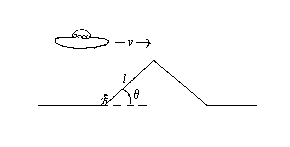Solution:

• Concepts:
Relativistic kinematics, the Lorentz transformation
• Reasoning:
The spaceship moves with uniform relativistic velocity vi with respect to the ground.  The ground and the spaceship are two inertial reference frames, moving with respect to each other.  We are given the space-time coordinates of two events in one reference frame.  We are asked to transform to another reference frame.
• Details of the calculation:
(a)  Let K' be the frame of the spaceship and K the frame of the observer on the ground.

 x0' x1' x2' x3'

=

 γ -γβ 0 0 -γβ γ 0 0 0 0 1 0 0 0 0 1

 x0 x1 x2 x3

.

 x0 x1 x2 x3

=

 γ γβ 0 0 γβ γ 0 0 0 0 1 0 0 0 0 1

 x0' x1' x2' x3'

.

Here (x0,x1,x2,x3) = (ct,x,y,z), β = v/c, γ = (1 - β2).
Assume that at t = t' = 0 the origins of the two coordinate systems coincide at r = r' = 0.
In K the archeologist's position 4-vector when he reaches the top is (cT, lcosθ, lsinθ, 0).
In K' it is (cT', x', y', 0), with
cT' = γcT - γβlcosθ,  x' = -γβcT + γlcosθ, y' = lsinθ.
T' = γ(T - (v/c2)lcosθ) is the time it takes the archeologist to get to the top in K'.
He moves a distance d' = [γ2(lcosθ -vT)2 + (lsinθ)2]½.
(b)  In K:  ∆s2 = c2∆t2 - |∆r|2 = c2T2 - (lcosθ)2 - (lsinθ)2 = c2T2 - l2.
In K':  ∆s2 = c2∆t'2 - |∆r'|2 = c2γ2(T - (v/c2)lcosθ)2 - γ2(lcosθ - vT)2 - (lsinθ)2
= γ2[c2T2 - 2βcTlcosθ + β2(lcosθ)2 - (lcosθ)2- (vT)2 + 2lvcosθT] - (lsinθ)2
= γ2[c2T2(1 - v2/c2) - (lcosθ)2(1 - β2)] - (lsinθ)2 = c2T2 - l2.
(c)  The proper time is calculated in the archeologist's rest frame.  He moves with constant velocity v0 = l/T with respect to an observer at rest at the foot of the pyramid.
∆τ = ∆t/γarch = T(1 - v02/c2)½ = (T2 - l2/c2)½ = ∆s/c.

#### Problem:

Consider three reference frames.  A meter stick is at rest in frame K2.  It is positioned on the x-axis, from x = 0 to x = 1m.  Frame K2 moves with velocity v = v2j in the positive y-direction with respect to frame K1.  Frame K3 moves with velocity v = v3i in the positive x-direction with respect to frame K1.
(a)  Find the velocity of the stick in K3.
(b)  Find the length of the stick in K3.
(c)  Find the angle θ the stick makes with the x-axis in K3.

Solution:

• Concepts:
Relativistic kinematics
• Reasoning:
We are asked to relate observations in an inertial frame K1 to observations in an inertial frame K3.
• Details of the calculation:
(a)  The stick is at rest in K2.  K2 moves with respect to K1 with speed v2 in the +y-direction.  So the stick moves with velocity v2j in K1.  It is oriented parallel to the x-axis, so its length in K1 is 1m.
K3 moves with velocity v3i with respect to K1.  The velocity of the stick in K3 can be found from the velocity addition formulas.
Assume that K' is moving with velocity vi with respect to K.  A particle moves in K with velocity u = dr/dt. The particle's velocity in K', u' = dr'/dt', is given by

u'|| = (u|| - v)/(1 - v∙u/c2)
u' =  u/(γ(1 - v∙u/c2))

where parallel and perpendicular refer to the direction of the relative velocity v.

Here v = v3i,  u|| = 0, u = v2j.
Therefore ux' = -v3,  uy' = v23 = (1 - v32/c2)½v2.

We can also proceed by transforming the coordinates of events.  Let us look at the positions of the left and the right end of the stick at t = 0 and at t = t1 in K1 and K3.
To transform the coordinates of an event from K1 to K3 we use the matrix equation below.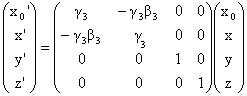Event: coordinates in K1 coordinates in K3 (1)  left edge at t = 0 (0, 0, 0, 0) (0, 0, 0, 0) (2)  right edge at t = 0 (0, L, 0, 0) (-γ3β3L, γ3L, 0, 0) (3)  left edge at t = t (ct, 0, v2t, 0) (γ3ct, -γ3β3ct, v2t, 0) (4)  right edge at t = t (ct, L, v2t, 0) (γ3ct-γ3β3L, -γ3β3ct+γ3L, v2t, 0)

From events 1 and 3 we find ux' = Δx'/Δt' = -γ3β3ct/γ3t = -β3c = -v3.
From events 1 and 3 we find uy' = Δy'/Δt' = v2t/γ3t = v23.
(b)  In K3 at t' = 0 the left edge of the stick is at x' = 0, y' = 0.
At t' = -γ3β3L/c the right edge of the stick is at x' = γ3L, y' = 0.
The stick travels with velocity u' = -v3i + (v23)j.  Therefore  at t' = 0 the right edge of the stick is at
x' = γ3L - v3γ3β3L/c,  y' = (v233β3L/c, or x' = γ3L(1 - β32) = L/γ3,  y' = β2β3L.
The length of the stick in K3 is (x'2 + y'2)½ = L(1 - β32 + (β2β3)2)½.
(c) tanθ' = y'/x' = β2β3γ3.

#### Problem:

A spaceship travels with velocity v = vi  with respect to a space station.  In the frame of the space station a linear structure of length L = 10 km moves with velocity u = (c/2)j in the positive y direction.  The structure lies in the xy-plane and makes an angle θ = 7.2o with the x axis?  When viewed from the spaceship, the structure is aligned with the x-axis.
(a)  What is the velocity v of the spaceship (magnitude and direction)?
(b)  What is the length of the structure in the frame of the spaceship'?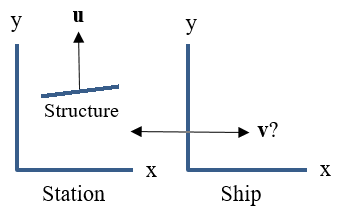Solution:

• Concepts:
Relativistic kinematics
• Reasoning:
We are asked to relate observations in different inertial frames.
• Details of the calculation:
(a)  Let us look at the positions of the left and the right ends of the structure at time 0 and at time t in frames K (space station) and K' (spaceship).  To transform the coordinates of an event from K to K' we use the matrix below.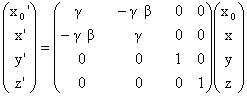Event: coordinates in K coordinates in K' (1)  left edge at t = 0 (0, 0, 0, 0) (0, 0, 0, 0) (2)  right edge at t = 0 (0, Lcosθ, Lsinθ, 0) (-γβLcosθ, γLcosθ, Lsinθ, 0) (3)  left edge at t = t (ct, 0, ut, 0) (γct, -γβct, ut, 0) (4)  right edge at t = t (ct,  Lcosθ , Lsinθ + ut, 0) (γct - γβLcosθ, -γβct + γLcosθ, Lsinθ + ut, 0)

From events 1 and 3 we find vx' = ∆x'/∆t' = -γβct/γt = -βc = -v.
From events 1 and 3 we find vy' = ∆y'/∆t' = ut/γt = u/γ.
In K' at t' = 0 the left edge of the stick is at x' = 0, y' = 0.
At t' = -γβLcosθ/c the right edge of the stick is at x' = γLcosθ, y' = Lsinθ.
The stick travels with velocity v' = -v i + (u/γ) j.
Therefore  at t' = 0 the right edge of the stick is at  x' = γLcosθ - vγβL/c,  y' = Lsinθ + (u/γ)γβL/c,
or x' = γL(cosθ - β2),  y' = L(sinθ + uv/c2).
For y' = 0 at t' = 0 we need sinθ + uv/c2 = 0,  v = -sinθ c2/u = -2 sin7.2o c = -0.25 c.
The ship is traveling in the negative x direction with velocity v = -0.25c i with respect to the station.

(b)  At t' = 0 the right edge of the stick is at x' = 0, y' = 0, the right edge of the stick is at
x' = γL(cosθ - β2), y' = 0, the length of the stick is L' = γL(cosθ - β2) = 0.96 L = 9.6 km.

#### Problem:

A spaceship has length 100 m in its own reference frame.  It travels at v = 0.95 c with respect to earth.  Suppose that the tail of the spaceship emits a flash of light.
(a)  In the reference frame of the spaceship, how long does it take the light to reach the nose?
(b)  In the frame of the earth, how long does it take the light to reach the nose?

Solution:

• Concepts:
Relativistic kinematics, the Lorentz transformation
• Reasoning:
We are asked to find the space-time coordinates of two events in one reference frame.
We are then asked to transform to another reference frame.
• Details of the calculation:
(a) In the frame of the spaceship it takes the light pulse t = 100 m/(3*108 m/s) = 333 ns to reach the nose.
(b) Let K be the frame of the spaceship and K' the frame of the earth.   Let K' travel with velocity v = -0.95 c i with respect to K', β = -0.95,  γ = (1 - β2)½ = 3.2.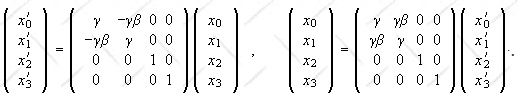in K in K' coordinates: x ct x' ct' Event 1: tail emits pulse 0 0 0 0 Event 2: nose receives pulse 100 100 -γβ100 + γ100 γ100 - γβ100

t' = (γ100 - γβ100)/c = (100 m)(3.2 + 3.04)/(3*108 m/s) = 2.08 μs.
or:
In the frame of the earth it takes the light 100 m(γ - γβ)/(3*108 m/s) = (100 m)(3.2 + 3.04)/(3*108 m/s) = 2.08 μs to reach the nose.

#### Problem:

(a)  Observer A is stationary in a laboratory on Earth's surface and observer B is in a laboratory moving at high velocity parallel to Earth's surface (neglect the curvature of the earth in the subsequent considerations) and parallel to the x axis.  Assume that the observers each witness two separate lightning strikes on the surface of the earth lying along the x axis.
(i)  Describe concisely a practical scheme whereby observer A can determine whether the two lighting strikes occurred simultaneously in her reference frame.
(ii)  Show that if observer A concludes from her observations that the strikes were simultaneous, observer B generally must conclude from his observations of the same events that the strikes are not simultaneous, thus demonstrating that simultaneity is a relative and not absolute concept.
(b)  Einstein was strongly inspired by Maxwell's theory of electromagnetism and viewed Galilean invariance for transformations between reference frames as being seriously flawed because it was inconsistent with Maxwell's theory.  The special theory of relativity removed this inconsistency.  Explain the meaning of the preceding two statements.

Solution:

• Concepts:
The Lorentz transformation, the simultaneity problem
• Reasoning:
We are asked to demonstrate that simultaneity is a relative and not absolute concept.
• Details of the calculation:
(a) A lightning strike at time t produces light, which travels through the atmosphere which speed c/n (n ~1) and sound, which travels through the atmosphere with speed vs.
Let tl be the time at which the light signal arrives at A and ts the time at which the sound signal arrives at A.
d = vs (ts - t),  d = (c/n)(tl - t).
Eliminate d and solve for t.
t = (vsts - (c/n)tl)/(vs - c/n).
If t is the same for both lightning strikes, then they occurred simultaneously in the frame of observer A.
In the fame of observer A the coordinates of event 1 are (ct1, x1, y1, z1) =  (0, d1, 0, 0).  (We set t = 0 at the time of the strike.)
The coordinates of event 2 are (ct2, x2, y2, z2) =  (0, d2, 0, 0).In the frame of observer B we have from the Lorentz transformation that the coordinates for event 1 are (ct1', x1', y1', z1') =  (-γβd1, γd1, 0, 0), and the coordinates for event 2 are (ct2', x2', y2', z21') =  (-γβd2, γd2, 0, 0).
Since d1 is not equal to d2, we have t1' not equal to t2'.  The two lightning strikes do not occur simultaneously in the frame of observer B.
(b)  Maxwell's equations predict that electromagnetic fields satisfy a wave equation in free space.  The equations do not contain any reference to a medium, and the propagation speed of any electromagnetic wave through the vacuum is c, regardless of the relative motion of the source and the observer.  The Galilean transformation predicts that the speed of electromagnetic waves depends on the relative motion of the source and the observer.
The special theory of relativity removes this inconsistency.

The postulates of special relativity are:
I. The laws of nature are the same in all inertial reference frames.
II. In vacuum, light propagates with respect to any inertial frame and in all directions with the universal speed c.  This speed is a constant of nature.

The second postulate of the special theory of relativity, however, goes beyond just Maxwell's equations.  Important parts of physics, such as the physics of elementary particles, cannot possibly be explained in terms of electrodynamics.

#### Problem:

(a)  Show that if two events are separated in space and time so that no signal leaving one event can reach the other, then there is an observer for whom the two events are simultaneous.
(b)  Show that the converse is also true: if a signal can get from one event to the other, then no observer will find them simultaneous.
(c)  Show if a signal can get from one event to the other, then there is an observer for whom the two events have the same space coordinates, i.e. for whom the two events "happen at the same place".

Solution:

• Concepts:
The Lorentz transformation of the position 4-vector, no signal can be transmitted with speed > c
• Reasoning:
Let ct, r be the coordinates of an event in a reference frame K and let ct', r' be the coordinates of the same event in a reference frame K' moving with velocity β = v/c with respect to K.
Then ct' = γ(ct - β·r),  r'|| =  γ( r||  - βct),   r' = r.
Here parallel and perpendicular refer to the direction of the relative velocity v,
and γ = (1 - β2) ≥ 1 , 0 ≤ β < 1.
• Details of the calculation:
(a)  Let ct1, r1 and ct2, r2 be the coordinates of events 1 and 2 in K and let
|r2 - r1| = |∆r| > c(t2 - t1) = c∆t.  Then no signal leaving event 1 can reach event 2 in K.
Choose a reference frame K' that moves with velocity β with respect to K where β points into the direction of ∆r.  Then c∆t' = γ(c∆t - β|∆r|) =  γ|∆r| (c∆t/ |∆r| - β)
Since   |∆r| > c∆t,  c∆t/|∆r| < 1 and we can choose β < 1 such that c∆t/|∆r| - β = 0.
Then c∆t' = 0, the two events are simultaneous in K'.

(b)  Let ct1, r1 and ct2, r2 be the coordinates of events 1 and 2 in K and let
|r2 - r1| = |∆r| < c(t2 - t1) = c∆t.  Then it is possible for a signal leaving event 1 to reach event 2 in K.
Choose a reference frame K' that moves with velocity β with respect to K where β points into the direction of ∆r.  Then c∆t' = γ(c∆t - β|∆r|) =  γ|∆r| (c∆t/|∆r| - β).
Since   |∆r| < c∆t,  c∆t/|∆r| >1 and we cannot choose β < 1 such that c∆t/|∆r| - β = 0.
Therefore c∆t' > 0 in every frame.  No observer will find the events simultaneous.
(c)  Let ct1, r1 and ct2, r2 be the coordinates of events 1 and 2 in K and let
|r2 - r1| = |∆r| < c(t2 - t1) = c∆t.  Then it is possible for a signal leaving event 1 to reach event 2 in K.
Choose a reference frame K' that moves with velocity β with respect to K where β points into the direction of ∆r.  Then r' = r = 0 and ∆r' =  γ(∆r  - βc∆t) = γ c∆t (∆r/(c∆t)  - β).
Since   |∆r| < c∆t,  (|∆r|/(c∆t) < 1 and we can choose β < 1 such that (∆r/(c∆t)  - β) = 0.
Then r' = 0, the two events have the same space coordinates.

#### Problem:

Two events "occur" at the same place in the laboratory frame of reference and are separated in time by 3 seconds.
(a)  What is the spatial distance between these events in a rocket frame moving with respect to the laboratory frame, in which the events are separated in time by 5 seconds?
(b)  What is the relative speed of the moving frame and the laboratory frame?

Solution:

• Concepts:
Relativistic kinematics, the Lorentz transformation
• Reasoning:
We are given the space-time coordinates of two events in one reference frame.  We are asked to transform to another reference frame.
• Details of the calculation:
(a)  Let K be the laboratory frame and K' be the rocket frame.
K                K'
c2∆t2 - ∆x2  = c2∆t'2 - ∆x'2.
c2(3 s)2 - 0   = c2(5 s)'2 - ∆x'2.
∆x'2 = c2(16 s2)  ∆x' = c(4 s) = 12*108 m
(b)  In the laboratory frame the two events "occur" at the same place.  In the rocket frame the laboratory place has moved 12*108 m in 5 s.  The relative speed of the two frames is therefore (12*108 m)/(5 s) = (4/5) c.
Alternatively:
dτ = dt/γ.  3 s = (5 s)/γ = (5 s)(1 - v2/c2)½.  v = (4/5) c.